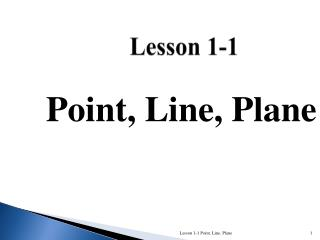DownloadDownload PresentationLesson 1-1

# Lesson 1-1

Télécharger la présentation## Lesson 1-1

- - - - - - - - - - - - - - - - - - - - - - - - - - - E N D - - - - - - - - - - - - - - - - - - - - - - - - - - -
##### Presentation Transcript

1. Lesson 1-1 Point, Line, Plane Lesson 1-1 Point, Line, Plane

2. Points • Point – a specific location in space • Points do not have actual size. • How to Sketch: Using dots • How to label: Use capital letters Never name two points with the same letter (in the same sketch). A B A C Lesson 1-1 Point, Line, Plane

3. Lines • Line- segment extending forever in opposite directions • Lines extend indefinitely and have no thickness or width. • How to sketch : using arrows at both ends. • How to name: several ways (1) small script letter – line n or n (2) any two points on the line - • Never name a line using three points – • 2 points make up a line n A B C Lesson 1-1 Point, Line, Plane

4. Planes • A plane is a flat surface that extends indefinitely in all directions. • How to sketch: Use a parallelogram (four sided figure) • How to name: 3 ways (1) Capital script letter – Plane M, M, Plane ABC (2) Any 3 non collinear points in the plane - Plane: ABC/ ACB / BAC / BCA / CAB / CBA A M B C Horizontal Plane Vertical Plane Other Lesson 1-1 Point, Line, Plane

5. Different planes in a figure: A B Plane ABCD Plane EFGH Plane BCGF Plane ADHE Plane ABFE Plane CDHG Etc. D C E F H G Lesson 1-1 Point, Line, Plane

6. Other planes in the same figure: Any three non collinear points determine a plane! Plane AFGD Plane ACGE Plane ACH Plane AGF Plane BDG Etc. Lesson 1-1 Point, Line, Plane

7. Coplanar Objects Coplanar objects (points, lines, etc.) are objects could be on the same plane. The plane does not have to be visible. Are the following points coplanar? A, B, C ? Yes Yes H, G, F, E ? E, H, C, B ? Yes A, G, F ? Yes Lesson 1-1 Point, Line, Plane

8. Non Coplanar • Points that could not be connected by a plane are said to be Non Coplanar points A C B F B C E G NO NO Lesson 1-1 Point, Line, Plane

9. Intersection of Figures The intersection of two figures is the list of all points that are shared by both figures. The intersection of two lines is a point. m Line m and line n intersect at point P. P n Continued……. Lesson 1-1 Point, Line, Plane

10. 3 Possibilities of Intersection of a Line and a Plane (1) Line passes through plane – intersection is a point. (2) Line lies on the plane - intersection is a line. (3) Line is parallel to the plane - no common points. Lesson 1-1 Point, Line, Plane

11. Collinear Points • Collinear points are points that lie on the same line. (The line does not have to be visible.) • A point lies on the line if the coordinates of the point satisfy the equation of the line. C B C A A B Collinear Non collinear Lesson 1-1 Point, Line, Plane

12. NoncollinearPoints • Noncollinear points are points that do no lie on the same line. C A B Non collinear Lesson 1-1 Point, Line, Plane

13. Quadrants/ Graphs I II ORIGIN X-Axis III IV Y-Axis Lesson 1-1 Point, Line, Plane

14. Postulates Definition: An assumption that needs no explanation. Examples: Postulate #1 • Through any two points there is • exactly one line. Postulate #3 • A line contains at least two points. • Through any three points, there is • exactly one plane. Postulate #2 • A plane contains at least three non collinear points. Postulate #1 Lesson 1-2: Segments and Rays

15. More Postulates Examples: • If two planes intersect, • then the intersecting is a line. • If two points lie in a plane, • then the line containing the two • points lie in the same plane. Lesson 1-2: Segments and Rays

16. Another Postulate Postulate #7: If 2 lines intersect then their intersection is exactly ONE POINT What is a Theorem? A theorem is a statement that can be PROVEN true. Lesson 1-2: Segments and Rays